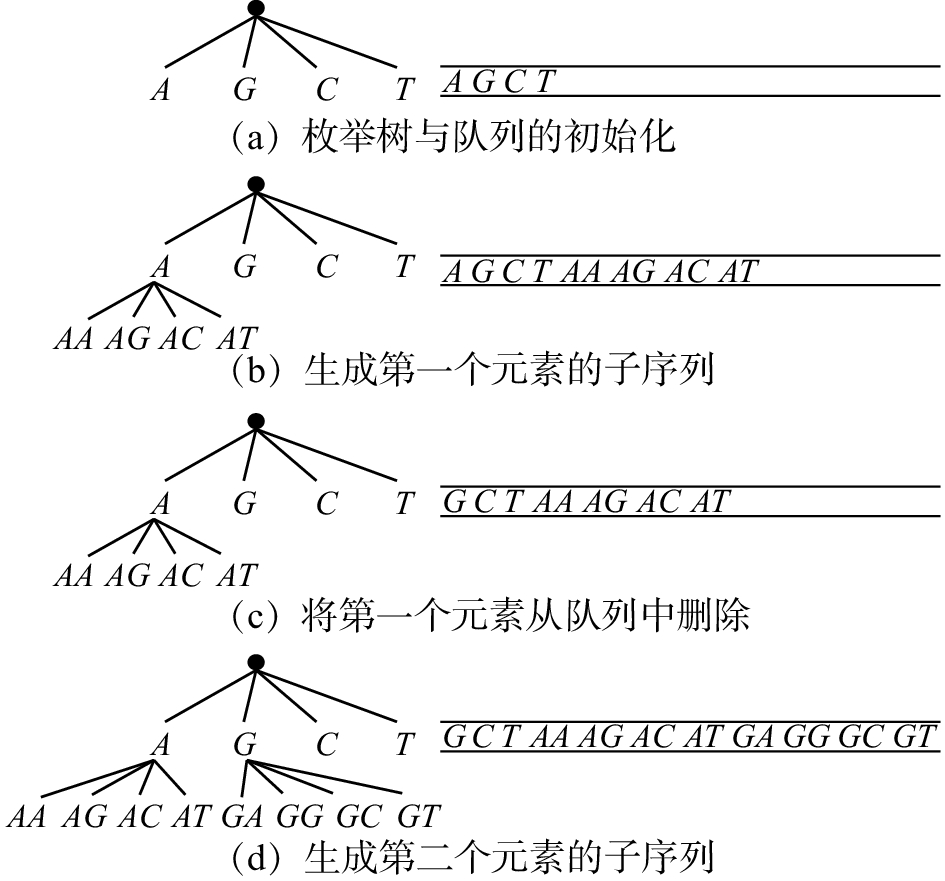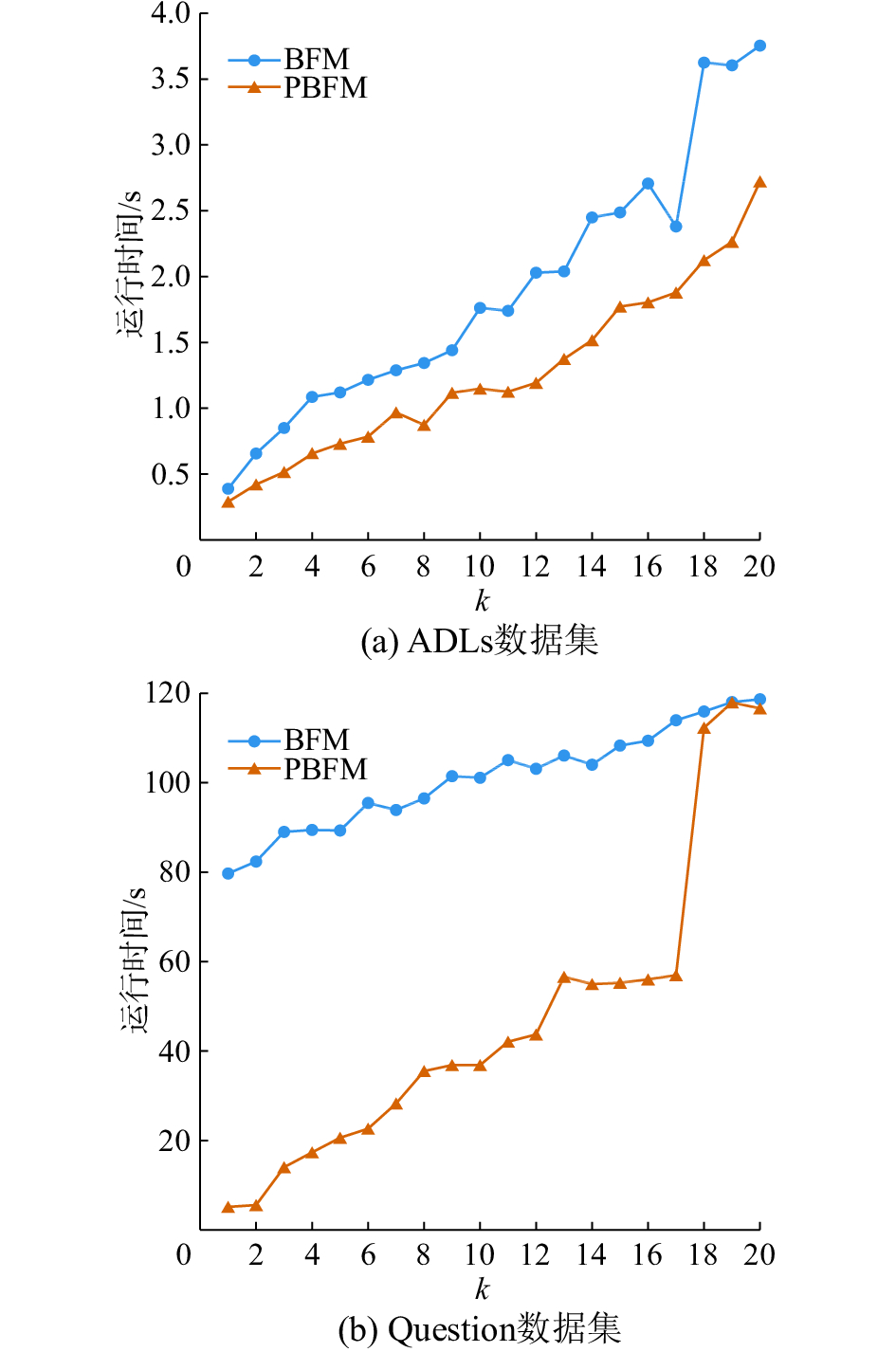﻿ 去冗余Top-k对比序列模式挖掘
«上一篇文章快速检索 高级检索

 智能系统学报2018, Vol. 13Issue (5): 680-686  DOI: 10.11992/tis.2017020190

### 引用本文JIANG Bing, GU Feiyang, HE Zengyou. Mining Top-k non-redundant distinguishing sequential patterns[J]. CAAI Transactions on Intelligent Systems, 2018, 13(5): 680-686. DOI: 10.11992/tis.201702019.### 文章历史

Mining Top-k non-redundant distinguishing sequential patterns
JIANG Bing, GU Feiyang, HE ZengyouSoftware School, Dalian University of Technology, Dalian 116621, China
Abstract: Distinct sequential patterns can be used to characterize different categories of datasets. In the field of bioinformatics, logistics management, and e-commerce, the comparison of sequential pattern has a wide range of applications. The goal of the Top-k distinguishing sequential pattern mining is to find k patterns with the highest contrast in a given data set. However, in the Top-k distinguishing sequential pattern mining, there is a redundancy problem with respect to the set of reported sequential patterns, which is not considered by the algorithm. Therefore, in this paper, a non-redundant Top-k distinguishing sequential pattern mining algorithm, breadth-first miner (BFM), is proposed based on breadth-first spanning tree. The redundancy problem is effectively solved using the BFM algorithm. Based on the BFM algorithm, a better algorithm, pruning breadth-first miner (PBFM), is proposed. Through the experimental analysis and comparison on the real data set, the validity of the algorithm is verified.
Key words: distinguishing sequential pattern    breadth-first    redundant sequential patterns    pattern mining    Top-k

1 相关工作

2 问题定义

 ${\rm{Sup}}(P,D) = \frac{{\left| {\left\{ {S \in D|P \subseteq S} \right\}} \right|}}{{\left| D \right|}}$ (1)

 ${\rm{CR}}(P,{D_ + },{D_ - }) = {\rm{Sup}}(P,{D_ + }) - {\rm{Sup}}(P,{D_ - })$表 1 含有两个类别的基因数据集 Tab.1 A gene data set with two classes

Top-k对比序列模式挖掘的目标是找出给定数据集中对比度最大的k个序列模式。表 2 表1中基因数据集的Top-5对比序列模式 Tab.2 Top-five discriminative sequential patterns from gene data set in table 1

1) $P'$ 是的 $P$ 子序列，即 $P' \subseteq P$

2) ${\rm{CR}}(P',{D_ + },{D_ - }) \geqslant {\rm{CR}}(P,{D_ + },{D_ - })$ ；则称模式 $P$ 是冗余对比序列模式。表 3 表1中基因数据集的Top-5去冗余对比序列模式 Tab.3 Top-five non-redundant distinguishing sequential patterns from gene data set in table 1表 4 符号及其含义 Tab.4 Symbols and their meaning
3 算法设计

3.1 广度优先的生成树算法

1) 根据给定的数据集 $D$ ，生成字母表 $\Sigma$

2) 创建Top-k集合L，设置集合 $L$ 的最小对比度阈值 $\min {\rm{TopkCR}} = 0$

3) 创建一个队列，将字母表中的每个元素放入队列中。

4) 对于队列的第一个元素，在其末尾分别与字母表中的每个元素连接，形成新的序列。

5) 计算每个新的序列P的支持度和对比度，如果 ${\rm{Sup}}(P,{D_ + }) \geqslant \min {\rm{TopkCR}}$ ，将P放入队列中，否则不放入队列中。

$\left| L \right| < k$ 时，在集合L中寻找序列P的子序列，若未找到子序列，将P加入集合L；若找到了子序列P，且P相对于P′不是冗余序列，则将P加入集合L，并更新集合 $L$ 的最小对比度阈值 $\min {\rm{TopkCR}}$ (若 ${\rm{CR}}(P,{D_ + },{D_ - }) \leqslant \min {\rm{TopkCR}}$ ，则min TopkCR = CR(P, D+, D))，否则不加入集合。

$\left| L \right| = k$ 时，如果 ${\rm{CR}}(P,{D_ + },{D_ - }) > \min {\rm{TopkCR}}$ ，在集合L中寻找序列P的子序列，若未找到子序列，用P替换集合L中对比度最小的序列；若找到了子序列P′，且P相对P′不是冗余序列，则用P替换集合L中对比度最小的序列，并更新集合L的最小对比度阈值 $\min {\rm{TopkCR}}$ (若此时集合中第二小的CR小于 ${\rm{CR}}(P,{D_ + },{D_ - })$ ，则将它设置为 $\min {\rm{TopkCR}}$ ，否则 $\min {\rm{TopkCR = }} {\rm{CR}}(P,{D_ + },{D_ - })$ )；否则不替换。

6) 将队列的第一个元素弹出。

7) 重复4)~6)，直到队列为空。Download: 图 1 生成树和队列的动态变化 Fig. 1 The dynamic change of spanning tree and queue

/*初始化*/

1）创建集合 $L$ ，设置集合 $L$ 的最小对比度阈值 $\min {\rm{TopkCR}} = 0$

2）创建队列，初始化队列queue为空；

3）计算出字母表 $\Sigma$ ，将字母表中的每个元素加入到queue中；

4）创建树的根节点,建立由根节点分别指向字母表中每个元素的连接，令min TopkCR = getMinTopkCR (L)；

5）对 $\Sigma$ 中的每一个元素 $e$ ，把 $e$ 添加到queue的第一个序列的末尾，组成新的序列P，如果 ${\rm{Sup}}(P,{D_ + }) \geqslant \min {\rm{TopkCR}}$ 则把 P加入到队列中；

6）如果 $\left| L \right| = k$ ，并且 ${\rm{CR}}(P,{D_ + },{D_ - }) > \min {\rm{TopkCR}}$ 那么在集合L中寻找P的子序列P′；

7）重复步骤 5）、6），直到对列为空。

3.2 剪枝策略

${\rm{CR}}(P,{D_ + },{D_ - }) = {\rm{Sup}}(P,{D_ + }) - {\rm{Sup}}(P,{D_ - })$ 可知， ${\rm{Sup}}(P,{D_ - }) \geqslant 0$ ，如果 ${\rm{Sup}}(P,{D_ + }) \leqslant \min {\rm{TopkCR}}$ 则CR $(P,{D_ + },{D_ - }) \leqslant \min {\rm{TopkCR}}$ ，序列P不是Top-k对比序列模式。对于任意一个模式P的超模式 $P'$ ，有 ${\rm{Sup}}(P',{D_ + }) \! \leqslant \! {\rm{Sup}}(P,{D_ + })$ ，因此 ${\rm{Sup}}(P',{D_ + })\! \leqslant \! \min {\rm{TopkCR}}$ 也成立。所以序列P′也不是Top-k对比序列模式。序列P不用放入队列，即把以序列P为根节点的子树从整体的生成树上剪枝。

/*初始化*/

1）创建集合L，设置集合L的最小对比度阈值 $\min {\rm{TopkCR}} = 0$

2）创建队列，初始化队列queue为空；

3）计算正例集 ${D_ + }$ 的字母表 $\Sigma$ ，将字母表中的元素加入到queue中；

4）创建树的根节点，建立由根节点分别指向字母表中每个元素的指针，令min TopkCR = getMinTopkCR (L)；

5）如果 ( ${\rm{Sup}}(e,{D_ + }) \leqslant \min {\rm{TopkCR}}$ ) 则从字母表 $\Sigma$ 中删除元素 $e$ ，对 $\Sigma$ 中的每一个元素 $e$ ，把e添加到队列的第一个序列的末尾，组成新的序列P

6）如果 $\left| L \right| = k$ ，并且 ${\rm{CR}}(P,{D_ + },{D_ - }) > \min$ TopkCR，则在集合 $L$ 寻找 $P$ 的子序列 $P'$

7）重复步骤 5）、6），直到队列为空。

4 实验结果 4.1 实验环境表 5 数据集的特征 Tab.5 The characteristics of the data sets
4.2 实验结果分析

1) 实验1(去冗余前后Top-k集合对比实验)表 6 去冗余前后Top-k序列模式集合的变化(ADLs数据集) Tab.6 The set of Top-k sequential patterns before and after eliminating redundancy(ADLs data set)表 7 去冗余前后Top-k序列模式集合的变化(E.Coli数据集) Tab.7 The set of Top-k sequential patterns before and after eliminating redundancy(E.Coli data set)表 8 去冗余前后Top-k序列模式集合的变化(UJI数据集) Tab.8 The set of Top-k sequential patterns before and after eliminating redundancy(UJI data set)

2) 实验2(加入剪枝策略前后的对比实验)

k的值分别从1取到20，比较算法1(BFM)和算法2(PBFM)运行的时间如图2所示。从图2中可以看出，随着k值的增加，两种算法的运行时间都在增长，但PBFM的运行时间明显少于BFM的运行时间。在ADLs数据集中，随着k值逐渐变大，这一区别越来越明显。在Question 数据集中，当k值较小时，这一区别较为明显。随着k值的增大，Top-k集合的最小对比度minTopkCR逐渐变小，当 $k \geqslant 18$ 时，PBFM算法中删除的元素个数较少，但PBFM算法运行的时间仍然少于BFM算法运行的时间。Download: 图 2 BFM和PBFM的效率对比 Fig. 2 Comparison of BFM and PBFM efficiencies
5 结束语

  ZHANG Minghua, KAO Ben, CHEUNG D W, et al. Mining periodic patterns with gap requirement from sequences[J]. ACM transactions on knowledge discovery from data, 2007, 1(2): 7. DOI:10.1145/1267066 (0)  PEI Jian, WANG Haixun, LIU Jian, et al. Discovering frequent closed partial orders from strings[J]. IEEE transactions on knowledge and data engineering, 2006, 18(11): 1467-1481. DOI:10.1109/TKDE.2006.172 (0)  YAN Xifeng, HAN Jiawei, AFSHAR R. CloSpan: mining: closed sequential patterns in large datasets[C]//Proceedings of the 3rd SIAM International Conference on Data Mining. San Francisco, USA, 2003: 166–177. (0)  JI Xiaonan, BAILEY J, DONG Guozhu. Mining minimal distinguishing subsequence patterns with gap constraints[J]. Knowledge and information systems, 2007, 11(3): 259-286. DOI:10.1007/s10115-006-0038-2 (0)  ZAKI M J. SPADE: an efficient algorithm for mining frequent sequences[J]. Machine learning, 2001, 42(1/2): 31-60. DOI:10.1023/A:1007652502315 (0)  YANG Hao, DUAN Lei, DONG Guozhu, et al. Mining itemset-based distinguishing sequential patterns with gap constraint[C]//Proceedings of the 20th International Conference on Database Systems for Advanced Applications. Hanoi, Vietnam, 2015: 39–54. (0)  杨皓, 段磊, 胡斌, 等. 带间隔约束的Top-k对比序列模式挖掘[J]. 软件学报, 2015, 26(11): 2994-3009. YANG Hao, DUAN Lei, HU Bin, et al. Mining Top-k distinguishing sequential patterns with gap constraint[J]. Journal of software, 2015, 26(11): 2994-3009. (0)  ZHENG Zhigang, WEI Wei, LIU Chunming, et al. An effective contrast sequential pattern mining approach to taxpayer behavior analysis[J]. World wide web, 2016, 19(4): 633-651. DOI:10.1007/s11280-015-0350-4 (0)  GHOSH S, FENG Mengling, NGUYEN H, et al. Risk prediction for acute hypotensive patients by using gap constrained sequential contrast patterns[J]. AMIA annual symposium proceedings, 2014, 2014: 1748-1757. (0)  FOURNIER-VIGER P, TSENG VS. Mining Top-K Non-redundant association rules[C]//Proceedings of the 20th International Symposium on Foundations of Intelligent Systems. Macau, China, 2012, 7661: 31–40. (0)  FOURNIER-VIGER P, TSENG V S. TNS: mining top-k non-redundant sequential rules[C]//Proceedings of the 28th Symposium on Applied Computing. Coimbra, Portugal, 2013: 164–166. (0)  KAMEYA Y, SATO T. RP-growth: Top-k mining of relevant patterns with minimum support raising[C]//Proceedings of the 12th SIAM International Conference on Data Mining. Anaheim, USA, 2012: 816–827. (0)  CHAN S, KAO B, YIP C L, et al. Mining emerging substrings[C]//Proceedings of 8th International Conference on Database Systems for Advanced Applications. Kyoto, Japan, 2003: 119–126. (0)  JI Xiaonan, BAILEY J, DONG Guozhu. Mining minimal distinguishing subsequence patterns with gap constraints[J]. Knowledge and information systems, 2007, 11(3): 259-286. DOI:10.1007/s10115-006-0038-2 (0)  DENG Kang, ZAIANE O R. An occurrence based approach to mine emerging sequences[C]//Proceedings of the 12th International Conference on Data Warehousing and Knowledge Discovery. Bilbao, Spain, 2010: 275–284. (0)  YU H H, CHEN Chunhao, TSENG V S. Mining emerging patterns from time series data with time gap constraint[J]. International journal of innovative computing information and control, 2011, 7(9): 5515-5528. (0)  WANG Xianming, DUAN Lei, DONG Guozhu, et al. Efficient mining of density-aware distinguishing sequential patterns with gap constraints[C]//Proceedings of the 19th International Conference on Database Systems for Advanced Applications. Bali, Indonesia, 2014: 372–387. (0)